## Sunday, December 27, 2020

### Electricity from Solar Energy: UNIZOR.COM - Physics4Teens - Electromagne...

Notes to a video lecture on http://www.unizor.com

Solar Energy → Electricity

Solar panels that produce electricity are not physically or chemically changed during this process. So, the only source of energy is the light, and the energy it carries is transformed by a solar panel into electricity, that is into movement of electrons.
In this lecture we will analyze how the energy of light forces the electrons to move.
The complete theory behind this process is based on principles of quantum mechanics and we will not be able to dive deep into this area, but a schematic description of the process will be presented.

Commercial solar panel are much more complex that might seem from the following explanation, but their engineering is not a subject of this lecture, which is only about the principle of generation of electricity from sun light.

Let's start with the most important component of a solar cell - silicon (Si).
Silicon is a metalloid, it has crystalline structure and its atoms have four valence electrons on the outer most orbit, next down orbit contains eight electrons and the inner most - two electrons.

Silicon is one of the most frequently occurring elements on Earth and, together with oxygen, forms molecules of silicon dioxide SiO2 - main component of sand.

All silicon atoms are neutral since the number of electrons in each one and the number of protons in each nucleus are the same and equal to 14.
Atoms are connected by their covalent bonds of valence electrons on the outer orbits into a lattice-like crystalline structure.

Picture below represents the crystalline structure of silicon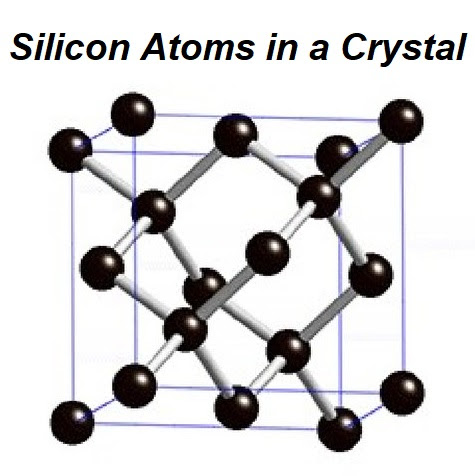For the purposes of this lecture we will represent inner structure of silicon atoms with only outer orbit of each atom with four valence electrons, as electrons on the inner orbits do not participate in the process of generating electricity from light.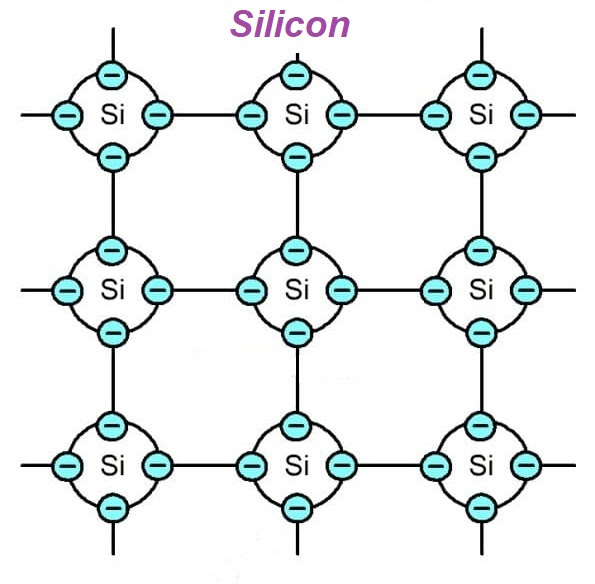The covalent bonds are quite strong, they do not easily release electrons. As a result, under normal conditions silicon is practically a dielectric.
However, if we excite the electrons sufficiently enough to break the covalent bonds, some electrons will move. For example, if we increase the temperature of a piece of silicon or put it under a bright sun light and measure its electrical resistance, we will observe the resistance diminishing.
That's why silicon and similar elements are called semiconductors.

While excited electrons of any semiconductor decrease its electrical resistance, they don't produce electromotive force because the material as a whole remains electrically neutral.
To build a solar cell that produces electricity under sun light we will introduce two kinds of "impurities" into crystalline lattice of silicon.
One is an element with five valence electrons on the outer most orbit, like phosphorus (P). When it's embedded into a crystalline lattice of silicon, one electron on that outer orbit of phosphorus would remain not attached to any neighboring atom through a covalent bond.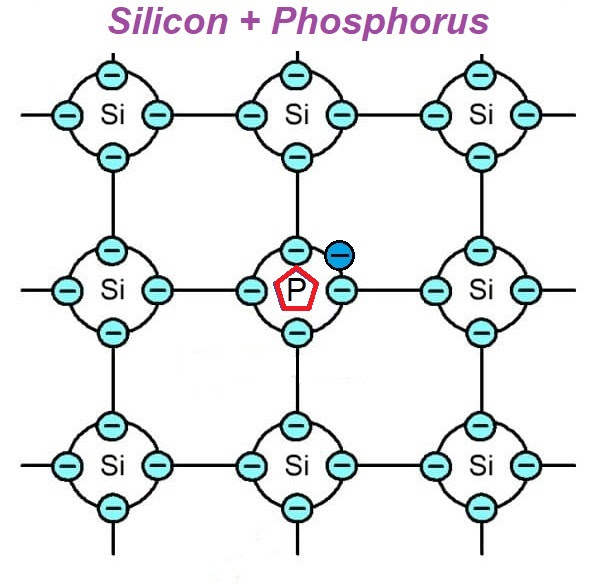This creates the possibility for this electron to start traveling, replacing other electrons and pushing them out, which are, in turn, push out others etc. Basically, we create as many freelance electrons as many atoms of phosphorus we add to silicon base.
The whole material is still neutral, but it has certain number of freelance electrons and the same number of stationary positive ions - those nuclei of phosphorus that lost electrons to freelancers.
Silicon with such addition is called n-type (letter n for negative).

Another type of "impurity" that we will add to silicon is an element with three valence electrons on the outer orbit, like boron (B).
When atoms of boron are embedded into a crystalline lattice of silicon, the overall structure of this combination looks like this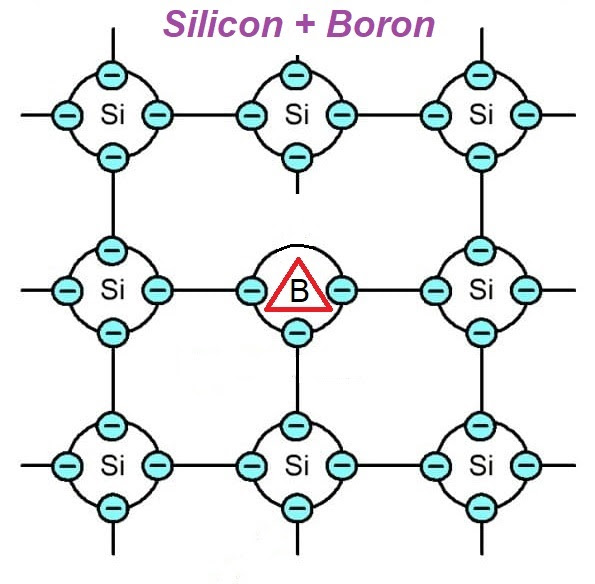In this case the lattice has a deficiency of an electron that is traditionally called a "hole". Existence of a "hole" opens the opportunity for neighboring valence electrons to fill it, thus creating a "hole" in another spot. These "holes" behave like positively charged particles traveling inside silicon with added boron inasmuch as negatively charged electrons travel in silicon with added phosphorus.
Silicon with such addition of boron is called p-type (letter p for positive).

Now imagine two types of "impure" silicon, n-type and p-type, contacting each other. In practice, it's two flat pieces (like very thin squares) on top of each other.
Let's examine what happens in a thin layer of border between these different types of material.

Initially, both pieces of material are electrically neutral with n-type having free traveling electrons and equal number of stationary positive nuclei of phosphorus inside a crystalline structure and with p-type having traveling "holes" and equal number of stationary negative nuclei of silicon inside a crystalline structure.

As soon as contact between these two types of material is established, certain exchange between electrons of the n-type material and "holes" of the p-type takes place in the border region called p-n junction. Electrons and "holes" in this border region combine, thus reconstituting the lattice.
This process is called recombination.

The consequence of this process of recombination is that n-type material near the border loses electrons, thus becoming positively charged, while the p-type gains the electrons, that is loses "holes", thus becoming negatively charged.

Eventually, the diffusion between n-type and p-type materials stops because negative charge of the p-type sufficiently repels electrons from the n-type. There will be some equilibrium between both parts.

If we introduce heat or bright sun light to electrons of n-type part, the diffusion will be longer and greater charge will be accumulated on both sides of the material - positive on the n-type and negative on the p-type.

Now the key point is to connect n-type side to p-type through some kind of electrical connection and extra electrons from the p_type will go to positively charged n-type, thus creating an electric current. This current is weak because only the diffusion between n-type and p-type in the border region is a contributing factor, but it's still the electric current.

Obviously, the more excited electrons in the n-type material are - the stronger diffusion inside the p-n junction is and the stronger current is produced. That's why, if sun light is used to excite the electrons, the more light falls on the n-type side - the stronger current is produced.

A pair of n-type and p-type materials form a cell. Since we are talking about using sun light to excite electrons, these cells are called solar cells or photovoltaic cells. Cells can be connected in a series increasing the produced electromotive force (voltage) or they can be connected in parallel to increase the electric current (amperage). Solar panels are sets of solar cells connected in series to increase the generated electromotive force (voltage).

Materials used to produce commercial solar cells can be different, not necessarily silicon with phosphorus or boron additions, but the main principle of using p-n junction between superconductors is the same for all.

## Sunday, December 20, 2020

### Chemical Energy to Electricity: UNIZOR.COM - Physics4Teens - Electromagn...

Notes to a video lecture on http://www.unizor.com

Chemical Energy → Electric Energy

To generate energy, the source components participating in the process should have more energy than the final components of the process. In case of generation of any form of energy from chemical energy we deal with chemical reaction, during which the inter-atomic energy of source components should exceed the inter-atomic energy of products of chemical reaction.

The simple form of such process is burning that produces heat. For example, burning methane CH4 in atmosphere that contains oxygen O2 is a chemical reaction described by
CH4 + 2O2 = CO2 + 2H2O
The inter-atomic energy of one molecule of methane and two molecules of oxygen must be greater than inter-atomic energy of a molecule of carbon dioxide and two molecules of water, otherwise there will not be any energy released as heat.

To generate electric energy from chemical we need the same type of inequality: the inter-atomic energy of primary components of the chemical reaction must be greater than inter-atomic energy of the resulting components.
The way how this excess of energy represented depends on the chemical reaction. In the process of burning the excess of energy is in a form of heat, in case of the generating of electricity the excess of energy is in a form of electric current that has certain voltage and amperage and, therefore, is a carrier of energy.

Transformation of chemical energy into electricity is typically occurring in batteries.
There are different types of batteries, and in this lecture we will mention a few with some details of how they work.

Consider a lead-acid battery used in many cars.
In a simplified way it has three major components: solid anode (negative electrode) made of lead Pb, solid cathode (positive electrode) made of lead dioxide PbO2 and liquid electrolyte in-between them containing sulfuric acid H2SO4 diluted in water H2O.To understand why electricity is generated by this device, let's look inside the atomic structure of its components and analyze what happens when there is a load (like a lamp) connected to its terminals.

Recall the classical planetary model of an atom.
There are protons and neutrons forming its nucleus and electrons circulating around this nucleus on different orbits.
Those electrons on outer most orbits are less attached to a host nucleus and many of them can fly around, potentially getting attached by other host nuclei.
This creates certain amount of "free" positively (with deficit of electrons) charged ions called cations and negatively (with access of electrons) charged ions called anions.

Analogously, molecules are also not completely stable and can lose atoms, if inter-atomic forces are not strong enough.
Applied to molecular structure of any substance, typical contents not only contains electrically neutral molecules, but also molecules with certain missing or extra atoms or electrons - ions.

Consider sulfuric acid inside a battery pictured above.
Structural composition of atoms of each molecule of this acid can be represented as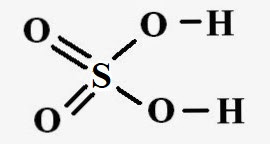Many of electrically neutral molecules of sulfuric acid H2SO4 are partially breaking losing a positive ions (nuclei with a single proton) of hydrogen but retaining their electrons, which can be represented as
H2SO4 → 2H + + SO42−
This can be explained by the fact that hydrogen nucleus contains only one proton, this is the lightest and electrically weakest nucleus and, being so light and volatile, it easily breaks its inter-atomic links with the main molecule of sulfuric acid. The resulting cations H + and anions SO42− are actually responsible for burning and corrosion caused by sulfuric acid. In batteries, however, these ions are responsible for producing electricity.
Here is how.

The negative anode of a lead-acid battery is made of lead (chemical symbol Pb), which, when going into chemical reactions, exhibits either 2 or 4 links to other atoms in the molecules.
The negative ion (anion) SO42−, produced during the breaking of the molecules of sulfuric acid, chemically reacts with led producing lead sulfate, and releasing two electrons left after ions of hydrogen broke free from the molecule of sulfuric acid as follows:
Pb+SO42− → PbSO4 + 2e
Structural composition of atoms of each molecule of lead sulfate can be represented as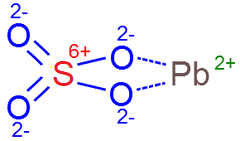Keep an eye on two electrons 2e produced at anode. They are produced on the surface of a lead anode and accumulated inside it up to a certain concentration that gives certain negative charge to an anode. These electrons will be the ones that produce the electric current, when some load (like a lamp) is connected to terminals of a battery.

Meanwhile, at the cathode terminal of a led-acid battery another reaction goes on between its main component lead dioxide PbO2, having the following structure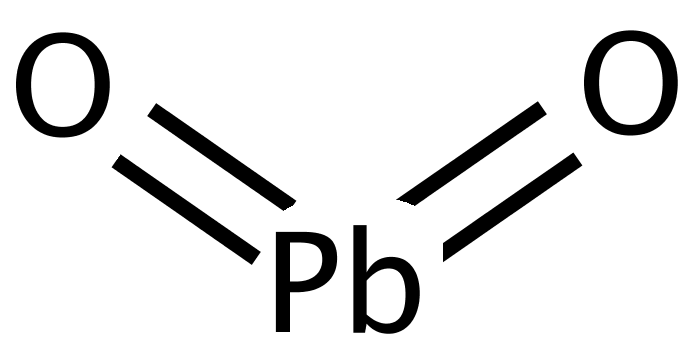and electrolyte that still contains unused positive ions of hydrogen 2H+ from the reaction on an anode and another pair of ions, positive 2H+ and negative SO42− of the sulfuric acid.

Now two reactions simultaneously go on at the surface of a cathode.
First of all, lead dioxide connects with negative ion of sulfuric acid, producing lead sulfate and releasing two negative ions of oxygen:
PbO2 + SO42−
→ PbSO4 + 2O

Secondly, remaining two positive ions of hydrogen from a molecule of sulfuric acid participating in the above reaction and two positive ions of hydrogen from the reaction on an anode meet two negative ions of oxygen from the above reaction, forming two molecules of water H2O with two electrons still missing:
4H+ + 2O → 2H2O2+

Concentration of these molecules of water missing two electrons creates a positive charge on the cathode terminal of a battery. When this concentration reaches certain level, positive ions of hydrogen cannot reach a cathode, ions of hydrogen are not readily breaking off the molecules of sulfuric acid and reaction stops at certain level of positive charge, unless we connect anode and cathode through some external electric load to allow accumulated on an anode electrons compensate missing electrons on a cathode.

So, we have a shortage of two electrons on a cathode terminal of a battery to form electrically neutral molecules of lead dioxide and water, but these two electrons can travel through an outside load between the terminals of a battery from its anode.
The overall reaction on a cathode looks like
PbO2 + 4H+ + SO42− + 2e
→ PbSO4 + 2H2O

As a result of these chemical reactions electrons released by a reaction on an anode travel to a cathode through external load, thus creating an electric current.
In an absence of an external load reactions on terminals of a battery continue until some limit of concentration of negative charge on an anode and positive charge on a cathode is reached, after which the reactions stops as further ionization is prevented by accumulated charges.

This is how chemical energy (inter-molecular links) is converted into electrical energy in a lead-acid battery.

The above described lead-acid battery is capable to work in reverse, to accumulate chemical energy, if external electromotive force is applied to its terminals. In this case all reactions go in reverse, that's how car battery is charged by alternator, when a car is in motion.
The overall picture of the lead-acid battery to discharge and charge is as follows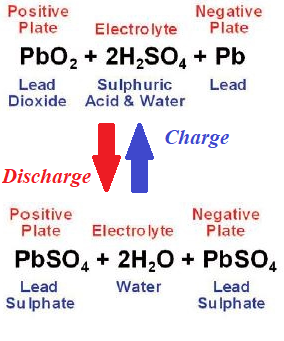## Monday, December 7, 2020

### Electricity from Kinetic Energy: UNIZOR.COM - Physics4Teens - Electromag...

Notes to a video lecture on http://www.unizor.com

Kinetic Energy → Electric Energy

The main principle used in converting kinetic energy into electric is the principle of electromagnetic induction.
Recall the Faraday's Law that defines the induced EMF as being proportional to a rate of changing the magnetic flux
EMF = −dΦ/dt

We can achieve a variable magnetic flux by either rotating the wire frame in the permanent magnetic field or rotating the magnetic field inside the wire frame. The corresponding designs were discussed earlier in this course.

In this lecture we will talk about how we can make a rotation of a rotor in the electric generator.

The simplest form of generating a rotational movement is if we already have some mechanical movement, so all we need is to transform one form of motion (usually, along some trajectory) into a rotational one.

As the first example of such purely mechanical device, consider a propeller.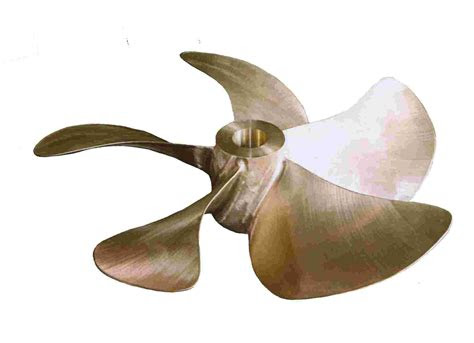It can be used to convert the flow of water in hydroelectric plants or the flow of wind through a wind turbine into a rotation. Once we have a rotational motion of the rotor in an electric generator, the rest goes along the previously described way of generation of electricity according to the laws of induction.
It can be a generation of alternating current, including three-phase one, or direct current. These were discussed in details in previous lectures.

In other cases we do not have already available motion that we can transform into a rotation, but we can artificially create one, using some other form of energy.
The common process thermal power station is to generate a flow of steam by heating the water or a flow of some kind of combustion gases. This can be done by using the burners that burn coal, oil or natural gas.
Another way of heating is to use nuclear energy to heat the water by controlling the chain reaction inside the radioactive core of a nuclear reactor.
In some cases the solar energy is used to heat the water to produce a flow of steam.
Rarely used types of heat are geothermal and ocean thermal sources.

In all these cases some kind of turbines are used to convert the flow of moving substance (water, air, gases, steam) into a rotation.
Steam turbines are just a more sophisticated type of propeller (or rather coaxial propellers), allowing to extract as much as possible energy from the steam flow.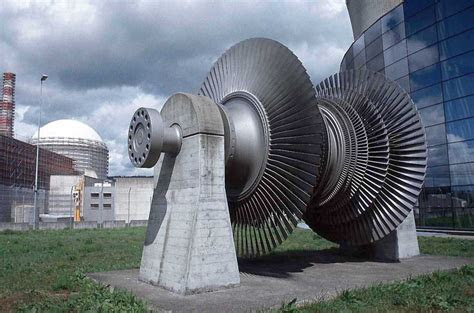Another form of generating electricity from heat and kinetic energy is internal combustion engines. The work of such engine results in a reciprocating motion of a piston, converted, using a connecting rod and a crankshaft, into rotation of a rotor of an electric generator that generates electricity.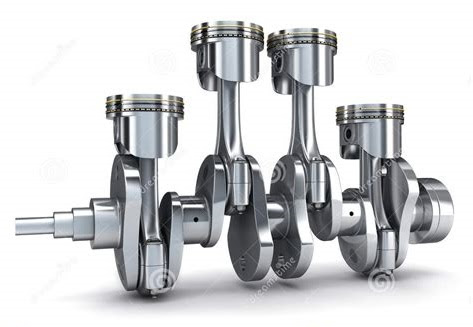In all the above cases the electricity is produced from kinetic energy of some substance, which is either readily available in nature (like water flowing along a river) or produced as a result of some process (like heating the water to produce a flow of steam).

## Sunday, November 22, 2020

### AC Power: UNIZOR.COM - Physics4Teens - Electromagnetism - AC Ohm's Law

Notes to a video lecture on http://www.unizor.com

AC Power

Power is a rate of work performed by some source of energy (like electric current) per unit of time. More precisely, it's a derivative of work performed by a source of energy, as a function of time, by time.

As we know from the properties of a direct electrical current, its power is
P = U·I = I²R = U²/R
where U is the voltage around a resistor of resistance R and I is the electric current going through this resistor.
All values in the above expression are constant for direct current.

Alternating current presents a problem of having the voltage and the current to be variable and dependent not only on the resistors, but also on the presence of inductors and capacitors in the circuit.

As described in the previous lecture, the alternating current in the circuit of a resistor, an inductor and a capacitor connected in a series to a generator of sinusoidal EMF equals to
I(t) = (E0 /Z)·sin(ωt+φ) =
= I0·sin(ωt+φ)

where impedance Z=√(XC−XL)²+R²,
tan(φ)=(XC−XL)/R.

Having expressions for generated EMF E(t)=E0·sin(ωt) and electric current in a circuit I(t)=I0·sin(ωt+φ), we can find an instantaneous power
P(t) = E(t)·I(t) =
= E0·I0·sin(ωt)·sin(ωt+φ)

When people talk about voltage or amperage in the AC circuit, they understand that these characteristics are variable and, to be more practical, they use effective voltage Eeff = E0 /2 and effective amperage Ieff = I0 /2 of the electric current. The usage of these characteristics allows to calculate the power consumed by a resistor-only circuit during a period [0,T] of time (for T significantly greater than one oscillation of a current) without integrating a variable function P(t)=E(t)·I(t) on interval [0,T], but just performing a multiplication of constants:
W[0,T] = Eeff · Ieff · T

Adding an inductor and a capacitor brings some complication because of a phase difference between EMF and a current. To express the power consumed by an AC circuit that includes a resistor, an inductor and a capacitor in terms of effective voltage and effective amperage, let's find the work performed by an electric current during a period of oscillation in terms of Eeff and Ieff and divide it by this period. The result would be an average power consumed by a circuit per time of one oscillation that we will call the effective power of a circuit.

The period of one oscillation with angular speed ω is T=2π/ω.
The instantaneous power consumption by a circuit is
P(t) = E(t)·I(t) =
= E0·sin(ωt)·I0·sin(ωt+φ)

The energy consumed by a circuit during one period of oscillation T=2π/ω equals to
W[0,T] = [0,T]P(t)·dt
where
P(t)=E0·sin(ωt)·I0·sin(ωt+φ)

We can simplify the product of two trigonometric functions to make it easier to integrate:
sin(x)·sin(y) =
= (1/2)·
[cos(x−y)−cos(x+y)]
Using this for x=ωt and y=ωt+φ, we obtain
sin(ωt)·sin(ωt+φ) =
= (1/2)·
[cos(φ)−cos(2ωt+φ)]

To find the power consumption during one period of oscillation T, we have to calculate the following integral
W[0,T] = [0,T]P(t)·dt
where period T=2π/ω and
P(t) = E0·I0·
·(1/2)·
[cos(φ)−cos(2ωt+φ)]

This integral can be expressed as a difference of two integrals
[0,T]E0·I0·(1/2)·cos(φ)·dt
which, considering cos(φ) is a constant for a given circuit, is equal to
E0·I0·(1/2)·cos(φ)·T =
= E0·I0·(1/2)·cos(φ)·2π/ω

and
[0,T]E0·I0·(1/2)·cos(2ωt+φ)·dt
which is equal to zero because integral of a periodical function cos(x) over any argument interval that equals to one or more periods equals to zero.
The same can be proven analytically
[0,T]cos(2ωt+φ)·dt =
= sin(2ωt+φ)/(2ω)|[0,T] =
= sin(2ω·2π/ω+φ)/(2ω) −
− sin(φ)/(2ω) =
=
[sin(4π+φ)−sin(φ)]/(2ω) = 0

Hence, the energy consumed by a circuit during one period of oscillation equals to
W[0,T] = E0·I0·(1/2)·cos(φ)·2π/ω
The average power consumption, that is the average rate of consuming energy that we will call effective power, equals to this amount of energy divided by time, during which it was consumed - one period of oscillation T=2π/ω:
Peff = W[0,T]/T =
= E0·I0·(1/2)·cos(φ)

Since Eeff = E0 /2 and Ieff = I0 /2, the last expression for power equals to
Peff = Eeff·Ieff·cos(φ)
where a phase shift φ depends on resistance and reactances of a circuit as follows
tan(φ) = (XC − XL) /R
XC = 1/(ωC) - capacitive reactance,
XL = ωL - inductive reactance,
R - resistance.
The above formula is derived for RLC-circuit that contains a resistor or resistance R, a capacitor of capacitance C and an inductor of inductance L
Let's analyze different circuits and their effective power consumption rate.

R-Circuit
R-circuit contains only a resistor. Therefore, both reactances XC and XL are zero and phase shift φ is zero as well. Since cos(0)=1, the effective power for this R-circuit is
Peff = Eeff·Ieff
which fully corresponds to a power for a circuit with a direct current running through it.

RC-Circuit
RC-circuit contains a resistor and a capacitor in a series. Reactance XL is zero.
Peff = Eeff·Ieff·cos(φ)
where tan(φ) = XC /R

RL-Circuit
RC-circuit contains a resistor and an inductor in a series. Reactance XC is zero.
Peff = Eeff·Ieff·cos(φ)
where tan(φ) = −XL /R
The negative values of tan(φ) is not important since function cos(φ) is even and cos(φ)=cos(−φ).

Interestingly, if our circuit contains a resistor, but a capacitor and an inductor are in resonance, that is XC=XL, the phase shift will be equal to zero, as if only a resistor is present in a circuit.

L-, C- and LC-Circuits
If no resistor is present in the circuit (assuming the resistance of wiring is zero), the denominator in the expression
tan(φ) = (XC − XL) /R
is equal to zero.
Therefore, the phase shift is φ=π/2=90°, cos(π/2)=0 and the power consumption is zero. So, only resistors contribute to a power consumption. Inductors and capacitors are not consuming any energy, they only shift the current phase relatively to a generated EMF. And, if an inductor and a capacitor are in resonance, there is no phase shift, they neutralize each other.

## Monday, November 16, 2020

### RLC Circuit Ohm Law: UNIZOR.COM - Physics4Teens - Electromagnetism - AC ...

Notes to a video lecture on http://www.unizor.com

RLC Circuit Ohm's Law

This lecture combines the material of the previous two ones dedicated to the Ohm's Law in alternating current circuits.
The first one was analyzing a circuit with a resistor and a capacitor.
The second one analyzed a circuit with a resistor and an inductor.
This lecture analyzes a circuit with all three elements - resistor, inductor and capacitor.

Consider a closed AC circuit with a generator with no internal resistance producing a sinusoidal electromotive force (EMF or voltage)
E(t)=E0·sin(ωt)(volt)
where E0 is a peak EMF produced by an AC generator,
ω is angular speed of EMF oscillations and
t is time.In this circuit we have a resistor with resistance R, an inductor with inductance L and a capacitor with capacitance C connected in series with EMF generator. This is RLC-circuit.
Since the circuit is closed, the electric current I(t) going through a circuit is the same for all components of a circuit.

Assume that the voltage drop on a resistor (caused by its resistance R) is VR(t), the voltage drop on an inductor (caused by its inductive reactance XL=ω·L) is VL(t) and the voltage drop on a capacitor (caused by its capacitive reactance XC=1/(ω·C)) is VL(t).

Since a resistor, an inductor and a capacitor are connected in a series, the sum of these voltage drops should be equal to a generated EMF E(t):
E(t) = VR(t) + VL(t) + VC(t)

As explained in the previous lectures the Ohm's Law localized around a resistor states that
I(t) = VR(t)/R or
VR(t) = I(t)·R

The inductance of an inductor L, voltage drop on this inductor caused by a self-induction effect VL(t), electromagnetic flux going through an inductor Φ(t) and electric current going through it I(t), as explained in the lecture "AC Inductors" of the chapter "Electromagnetism - Alternating Current Induction", are in a relationship
VL = dΦ/dt = L·dI(t)/dt

The capacity of a capacitor C, voltage drop on this capacitor VC(t) and electric charge accumulated on its plates QC(t), according to a definition of a capacitance C, are in a relationship
C = QC(t)/VC(t) or
VC(t) = QC(t)/C

We have expressed both voltage drops VR(t) and VL(t) in terms of an electric current I(t):
VR(t) = I(t)·R
VL(t) = L·I'(t)
Since an electric charge QC(t) is also involved to express the voltage drop on a capacitor, we will use this electric charge as a main variable, using the definition of an electric current as a rate of change of electric charge
I(t) = QC'(t) and
I'(t) = QC"(t)

Now all three voltage drops can be expressed as functions of QC(t) and, equating their sun to a generated EMF E(t), we can the following differential equation
E0·sin(ωt) = QC'(t)·R +
+ L·QC"(t) + QC(t)/C =
= L·QC"(t) + R·QC'(t) +
+ (1/C)·QC(t)

As we know, capacitive and inductive reactance are functionally equivalent to resistance, and they all have the same unit of measurement - ohm. Therefore, it's convenient, instead of capacitance C and inductance L, to use corresponding reactance XC=1/(ω·C) and XL=ω·L.
So, we will substitute
C = 1/(ω·XC)
L = XL

Substitute QC(t)=y(t) for brevity. Then our equation looks like
(XL/ω)·y"(t) + R·y'(t) +
+ ω·XC·y(t) = E0·sin(ωt)

Solving this equation for y(t)=QC(t) and differentiating it by time t, we will obtain the expression for an electric current I(t) in this circuit as a function of all given parameters and time.

First of all, let's find a particular solution of this differential equation.
The form of the right side of this equation prompts to look for a solution in trigonometric form
y(t) = F·sin(ωt) + G·cos(ωt)
Then
y'(t) =
= ω·
[F·cos(ωt)−G·sin(ωt)]
y"(t) =
= −ω²·
[F·sin(ωt)+G·cos(ωt)] =
= −ω²·y(t)

Using this trigonometric representation of potential solution y(t), the left side of our equation is
(XL/ω)·y"(t) + R·y'(t) +
+ ω·XC·y(t) =
= −(XL/ω)·ω²·y(t) + R·y'(t) +
+ ω·XC·y(t) =
= −ω·XL·y(t) + R·y'(t) +
+ ω·XC·y(t) =
= R·y'(t) + ω·(XC−XL)·y(t) =
= R·ω·
[F·cos(ωt)−G·sin(ωt)] +
+ ω·(XC−XL
[F·sin(ωt)+
+G·cos(ωt)
] =
= ω·
[(XC−XL)·F−R·G]·sin(ωt)+
+ω·
[R·F+(XC−XL)·G]·cos(ωt)

Since this is supposed to be equal to E0·sin(ωt), we have the following system of two linear equations with two variables F and G
ω·[(XC−XL)·F−R·G] = E0
ω·[R·F+(XC−XL)·G] = 0
or
(XC−XL)·F−R·G = E0/ω
R·F+(XC−XL)·G = 0

Determinant of the matrix that defines this system is
(XC−XL)²+R².
It's always positive and usually is denoted as
Z² = (XC−XL)²+R²
The value Z is called the impedance of a circuit and, as we will see, plays the role of a resistance for an entire RLC-circuit.

This system of two linear equations with two variables has a solution:
F = (E0/ω)·(XC−XL)/
G = −(E0/ω)·R/

Consider two expressions that participate in the above solutions F and G:
(XC−XL)/ and R/
Since Z² = (XC−XL)²+R², we can always find an angle φ such that
(XC−XL)/Z = sin(φ) and
R/Z = cos(φ)

Using this, we express the solutions to the above system as
F = (E0/(ω·Z))·sin(φ)
G = −(E0/(ω·Z))·cos(φ)

Now the solution of the differential equation for y(t)=QC(t) that we were looking for in a format
y(t) = F·sin(ωt) + G·cos(ωt)
looks like
y(t)=(E0/(ω·Z))·sin(φ)sin(ωt) −
− (E0/(ω·Z))·cos(φ)cos(ωt) =
= −(E0/(ω·Z))·cos(ωt+φ)

As we noted before, differentiation of this function gives the electric current in the circuit I(t):
I(t) = y'(t) =
= (E0 /(ω·Z))·ω·sin(ωt+φ) =
= (E0 /Z)·sin(ωt+φ) =
= I0·sin(ωt+φ)

where I0=E0 /Z is an equivalent of the Ohm's Law for an RLC-circuit.

Similar relationship exists between effective voltage and effective current
Ieff = I0 /2 =
= E0 /(√2·Z) = Eeff /Z

Here impedance Z, defined by resistance R, inductive reactance XL and capacitive reactance XC as
Z = √(XC−XL)²+R²
plays a role of a resistance in the RLC-circuit.

There is a phase shift φ of the electric current oscillations relative to EMF. It is also defined by the same characteristics of an RLC-circuit:
(XC−XL)/Z = sin(φ)
R/Z = cos(φ)
Hence
tan(φ) = (XC−XL)/R
This makes phase shift positive or negative depending on the values of capacitive and inductive reactance.
If XC is greater than XL, the phase shift is positive, if the reverse is true, the phase shift is negative.
If the values of reactance are the same, that is XC=XL, there is no phase shift. From definition of reactance, it happens when
1/(ω·C) = ω·L or
1/(L·C) = ω² or
L·C = 1/ω²
This relationship between inductance, capacitance and angular speed of EMF oscillation is call resonance.

Expressions for electric current
I(t) = (E0 /Z)·sin(ωt+φ) =
= I0·sin(ωt+φ)

in RLC-circuit, where impedance Z=√(XC−XL)²+R² and tan(φ)=(XC−XL)/R, correspond to analogous formulas for R-, RC- and RL-circuits presented in the previous lectures. All it takes is to set the appropriate value of capacitance or inductance to zero.

## Tuesday, November 10, 2020

### Ohm's Law for RL_Circuit: UNIZOR.COM - Physics4Teens - Electromagnetism ...

Notes to a video lecture on http://www.unizor.com

RL Circuit Ohm's Law

Consider a closed AC circuit with a generator with no internal resistance producing a sinusoidal electromotive force (EMF or voltage)
E(t)=E0·sin(ωt)(volt)
where E0 is a peak EMF produced by an AC generator,
ω is angular speed of EMF oscillations and
t is time.
In this circuit we have a resistor with resistance R and an inductor with inductance L connected in series with EMF generator. This is RL-circuit.
Since the circuit is closed, the electric current I(t) going through a circuit is the same for all components of a circuit.

Assume that the voltage drop on a resistor caused by its resistance is VR(t) and the voltage drop on an inductor caused by self-induction effect is VL(t). Since a resistor and an inductor are connected in a series, the sum of these voltage drops should be equal to a generated EMF E(t):
E(t) = VR(t) + VL(t)

As explained in the previous lecture for an R-circuit, the Ohm's Law localized around a resistor states that
I(t) = VR(t)/R or
VR(t) = I(t)·R

The inductance of an inductor L, voltage drop on this inductor caused by a self-induction effect VL(t), electromagnetic flux going through an inductor Φ(t) and electric current going through it I(t), as explained in the lecture "AC Inductors" of the chapter "Electromagnetism - Alternating Current Induction", are in a relationship
VL = dΦ/dt = L·dI(t)/dt

We have expressed both voltage drops VR(t) and VL(t) in terms of an electric current I(t):
VR(t) = I(t)·R
VL(t) = L·I'(t)

Now we can substitute them into a formula for their sum being equal to a generated EMF
E(t) = VR(t) + VL(t) =
= I(t)·R + I'(t)·L

Since E(t)=E0·sin(ωt) we should solve the following differential equation to obtain function I(t)
E0·sin(ωt) = I(t)·R + I'(t)·L

Let's divide this equation by L and use simplified notation for brevity:
y(t) = I(t)
a = R/L
b = E0/L
Then our equation looks simpler
y'(t)+a·y(t) = b·sin(ωt)

This exact differential equation was solved in the previous lecture dedicated to RC-circuit, notes for that lecture contain detail analysis of its solution
y(t) = −b·cos(ωt+ψ)/√(a²+ω²) + K
where phase shift ψ=arctan(a/ω) and K is a constant that can be determined by initial condition.

Using original notation,
I(t) = −(E0/L)·cos(ωt+ψ)/
/(R/L)²+ω² + K =
= −E0·cos(ωt+ψ)/
/R²+(ω·L)² + K =
= −E0·cos(ωt+ψ)/R²+XL² + K

where XL=ω·L is inductive reactance of an inductor - a characteristic of an inductor functionally equivalent to a resistance for a resistor and measured in the same units - ohm and
tan(ψ) = a/ω = R/(L·ω) = R/XL

Let's apply some Trigonometry to simplify the above formula.
−cos(ωt+ψ) =
= −sin((π/2)−ωt−ψ) =
= −sin((π/2)−ψ−ωt) =
= sin(ωt−((π/2)−ψ)) =
= sin(ωt−φ)

where φ=(π/2)−ψ and, therefore, tan(φ)=1/tan(ψ)=XL/R.

Using phase shift φ, the equation for the current I(t) looks like
I(t) = E0·sin(ωt−φ)/R²+XL² + K
where tan(φ)=XL/R.

The value of a constant K can be defined by initial conditions. Since we don't really know these conditions (like what is the value of a current at some moment in time), traditionally this constant is assigned the value of zero, motivating it by the fact that, if the generated EMF is oscillating between its minimum and maximum of the same magnitude with different signs, the current also will be "symmetrical" relative to zero level, which requires the value K=0

The final version of the current in this RL-circuit is
I(t) = E0·sin(ωt−φ)/R²+XL² =
= I0·sin(ωt−φ)

where I0 = E0/R²+XL²

The last issue is to analyze the effective current in this RL-circuit. Since for sinusoidal oscillations the effective current is by √2 less than peak amperage, the effective current is
Ieff = I0 /2 =
= E0 /(√XL²+R²·√2) =
= Eeff /XL²+R²

This is the Ohm's Law for effective voltage and amperage in RC-circuit.

It's important to notice that in the absence of a resistor (that is, R=0) the formula for I(t) transforms into
I(t) = I0·sin(ωt−φ)
where peak amperage I0=E0/XL and phase shift φ=arctan(∞)=π/2, which corresponds to results obtained in a lecture "AC Inductors" of the "Alternating Current Induction" chapter of this course dedicated to only an inductor in the AC circuit, taking the current as given and deriving the EMF.

## Saturday, November 7, 2020

### Ohm's Law for R- and RC-Circuits: UNIZOR.COM - Physics4Teens - Electromagnetism - AC O...

Notes to a video lecture on http://www.unizor.com

R and RC Circuit Ohm's Law

In this chapter we will examine different aspects of the Ohm's Law as they occur in different alternating current (AC) circuits.
Four different types of AC circuits will be considered in this and subsequent lectures:
(a) R-circuit that contains only a resistor;
(b) RC-circuit that contains a resistor and a capacitor;
(c) RL-circuit that contains a resistor and an inductor;
(d) RLC-circuit that contains a resistor, an inductor and a capacitor.
The first two are subject to this lecture.

R-circuit

Consider a closed AC circuit with a generator with no internal resistance producing a sinusoidal electromotive force (EMF or voltage)
E(t)=E0·sin(ωt)(volt)
where E0 is a peak EMF produced by an AC generator,
ω is angular speed of EMF oscillations
and t is time.
In this circuit there is only a resistor with resistance R(ohm).

During any infinitesimal time interval the electric current going through a circuit depends only on the generated EMF and a resistance of a circuit components. Since the only resistive component is a resistance R, the electric current at any moment of time will obey the Ohm's Law for direct current.

Therefore the electric current I(t) in this R-circuit is
I(t) = E(t)/R = E0·sin(ωt)/R =
= (E0/R)·sin(ωt) = I0·sin(ωt)

where I0 = E0/R is a peak current.
Now we can express the Ohm's Law for peak voltage and peak amperage in the R-circuit as
I0 = E0 /R

Since in many cases we are interested in effective voltage and effective electric current, and knowing that
Eeff = E0 /2 and Ieff = I0 /2,
the Ohm's Law for effective voltage and effective amperage in the R-circuit can be expressed in a form
Ieff = Eeff /R

RC-circuit

Let's add a capacitor with capacity C (farad) to R-circuit, connecting it in series with a resistor. This is RC-circuit.
As we know, alternating current goes through a capacitor, so we have a closed circuit of resistor R and capacitor C connected in a series with EMF generator that produces sinusoidal voltage E(t)=E0·sin(ωt).
The electric current I(t) going through a circuit is the same for all components of a circuit.

Assume that the voltage drop on a resistor is VR(t) and the voltage drop on a capacitor is VC(t). Since a resistor and a capacitor are connected in a series, the sum of these voltage drops should be equal to a generated EMF E(t):
E(t) = VR(t) + VC(t)

As explained above for an R-circuit, the Ohm's Law localized around a resistor states that
I(t) = VR(t)/R or
VR(t) = I(t)·R

The capacity of a capacitor C, voltage drop on this capacitor VC(t) and electric charge accumulated on its plates QC(t), according to a definition of a capacity, are in a relationship
C = QC(t)/VC(t) or
VC(t) = QC(t)/C

At the same time, by definition of the electric current, the electric current going through a capacitor is just a rate of change of electrical charge on its plates, that is, a derivative of an accumulated electric charge by time:
I(t) = Q'C(t)

This is the same electric current that goes through a resistor, since the circuit is closed. Therefore, we can substitute this expression of an electric current into a formula for a voltage drop on a resistor
VR(t) = Q'C(t)·R

We have expressed both voltage drops VR(t) and VC(t) in terms of an electric charge QC(t) accumulated on the plates of a capacitor:
VR(t) = Q'C(t)·R
VC(t) = QC(t)/C

Now we can substitute them into a formula for their sum being equal to a generated EMF
E(t) = VR(t) + VC(t) =
= Q'C(t)·R + QC(t)/C

Since E(t)=E0·sin(ωt) we should solve the following differential equation to obtain function QC(t)
E0·sin(ωt) =
= Q'C(t)·R + QC(t)/C

Let's divide this equation by R and use simplified notation for brevity:
y(t) = QC(t)
a = 1/(R·C)
b = E0/R
Then our equation looks simpler
y'(t)+a·y(t) = b·sin(ωt)

Let's discuss how to solve (integrate) this differential equation.
Recall the formula for a derivative of a product of two functions
(x(t)·y(t))' =
= x(t)·y'(t) + x'(t)·y(t)

Let's find such function x(t) that, if we use it as a multiplier of our differential equation, the left side will look like a complete derivative of x(t)·y(t).

Multiplied by this function x(t), our equation looks like this:
x(t)·y'(t)+a·x(t)·y(t) =
= b·x(t)·sin(ωt)

Let's find function x(t) such that a·x(t) is x'(t). Then the left side of the equation will be equal to (x(t)·y(t))'.

Obvious choice for such function is ea·t. It's an intelligent guess, but it can be determined analytically.
Indeed,
a·x(t) = x'(t)
a·x(t) = dx/dt
dt = dx/x(t)
d(a·t) = dln(x(t))
a·t = ln(x(t)) + K1
x(t) = K2·ea·t
Here K1 and K2 are any constants, so let's use K2=1.
Then x(t)=ea·t.

Now the equation takes the following form
ea·t·y'(t)+a·ea·t·y(t) =
= b·ea·t·sin(ωt)

which is equivalent to
[ea·t·y(t)]' = b·ea·t·sin(ωt)

The above equation should be integrated to get ea·t·y(t) and, finally y(t).

The indefinite integral of the left side is ea·t·y(t).

The indefinite integral of the right side can be found straight forward using Euler formula
cos(φ)+i·sin(φ) = ei·φ
and following from it expressions for trigonometric functions
cos(φ) = (ei·φ+e−i·φ)/2
sin(φ) = (ei·φ−e−i·φ)/(2i)

The result of integration of the right side (without multiplier b) is
ea·t·sin(ωt)·dt =
=
[ea·t/(a²+ω²)]·
·
[a·sin(ωt)−ω·cos(ωt)] + K3
(K3 is any constant)

Therefore,
ea·t·y(t) = b·[ea·t/(a²+ω²)]·
·
[a·sin(ωt)−ω·cos(ωt)] + K3

Reducing by ea·t both sides, the expression for y(t)=QC(t) looks like
y(t) = [b/(a²+ω²)]·
·
[a·sin(ωt)−ω·cos(ωt)] + K4

Consider two constants a/√(a²+ω²) and ω/√(a²+ω²). It is convenient to represent them as sin(φ) and cos(φ) correspondingly for some angle φ=arctan(a/ω).
Then
a·sin(ωt)−ω·cos(ωt) =
= √(a²+ω²)·
·
[sin(φ)·sin(ωt)−cos(φ)·cos(ωt)]

The last expression equals to
−√(a²+ω²)·cos(ωt+φ)
and our equation for y(t) looks like
y(t) = −b·cos(ωt+φ)/√(a²+ω²) +
+ K4

Since y(t) is the electric charge QC(t) accumulated on the plates of a capacitor, its derivative is an electric current in the circuit:
I(t) = b·ω·sin(ωt+φ)/√(a²+ω²)

Let's restore this equation to original constants
a = 1/(R·C)
b = E0/R
The factor at sin(ωt+φ) in the equation above then is
b·ω/√(a²+ω²) =
= E0·ω/(R·√1/(R·C)²+ω²) =
= E0/(√1/(ωC)²+R²) =
= E0/(√XC²+R²)

where XC=1/(ωC) was defined in one of the previous lectures as reactance of a capacitor (or capacitive reactance).

The angle φ=arctan(a/ω) in terms of original constants is
φ=arctan(1/(R·C·ω)) =
= arctan (XC / R)

This angle is called phase shift of the current from the voltage.

Now we have a formula for an electric current in the RC-cirucit that connects generated EMF, resistance of a resistor and capacity of a capacitor
I(t) = E0·sin(ωt+φ)/√XC²+R² =
= I0·sin(ωt+φ)

where I0=E0/√XC²+R² is the peak amperage of the electric current.
This is the Ohm's Law for RC-circuit.

The expression XC²+R² plays in this case the same role as a resistance in case of direct current circuits.

It is important that there is a phase shift φ of the oscillations of an electric current relatively to oscillation of generated EMF.

The last issue is to analyze the effective current in this RC-circuit. Since for sinusoidal oscillations the effective current is by √2 less than peak amperage, the effective current is
Ieff = I0 /2 =
= E0 /(√XC²+R²·√2) =
= Eeff /XC²+R²

This is the Ohm's Law for effective voltage and amperage in RC-circuit.

It's important to notice that in the absence of a resistor (that is, R=0) the formula for I(t) transforms into
I(t) = I0·sin(ωt+φ)
where peak amperage I0=E0/XC and phase shift φ=arctan(∞)=π/2, which corresponds to results obtained in a lecture "AC Capacitors" of the "Alternating Current Induction" chapter of this course dedicated to only a capacitor in the AC circuit.

## Monday, October 26, 2020

### Problems on LC Circuits: UNIZOR.COM - Physics4Teens - Electromagnetism -...

Notes to a video lecture on http://www.unizor.com

Problems on LC Circuit

Problem A

Consider a circuit that contains an AC generator, an inductor of inductance L and a capacitor of capacity C in a series.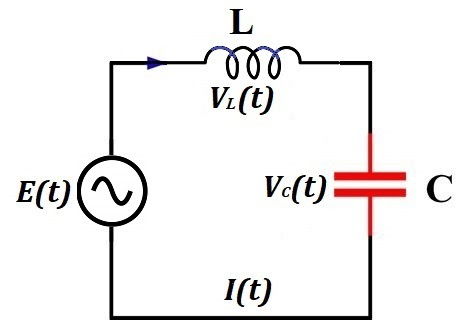Generated EMF has frequency f=50Hz and effective voltage Eeff=220V (volt).
The effective AC current is Ieff=5A (ampere).
The capacitance of a capacitor is C=10μF (microfarad).
Find inductance L (henry) of an inductor?

Solution

Let's start with an expression for the AC current in the LC circuit in terms of generated EMF and reactance of the capacitor and inductor.

EDF generated by a source of electricity
E(t) = E0·sin(ωt)
where
E0 is a peak voltage on the terminals of a generator,
ω=2πf is an angular velocity of a rotor in radians per second, where f is a frequency of generated EMF in number of cycles per second.

Alternating electric current in the circuit
I(t) = I0·cos(ωt)
where
I0 = E0/(XC−XL) is peak amperage,
XC = 1/(ω·C) is capacitive reactance,
XL = ω·L is inductive reactance

Effective voltage and effective amperage are less than, correspondingly, peak voltage and peak amperage by 2.
Therefore, from the expression for AC current follows
I(t) = I0·cos(ωt) =
= E0·cos(ωt)/(XC−XL)

Ieff = I0/√2 =
= E0/
[2(XC−XL)] =
= Eeff/(XC−XL)

The unknown in this expression is XL that can be found:
XC−XL = Eeff/Ieff
XL = XC−Eeff/Ieff

Since XC=1/(ω·C) and XL=ω·L L·ω = 1/(ω·C) − Eeff/Ieff
L = 1/(ω²·C) − Eeff/(ω·Ieff)

Substituting
ω=2πf=2π·50Hz=314(1/sec)
C=10μF=10−5F
Eeff=220V
Ieff=5A
into above expression for L, we obtain (rounded to 0.001)
L= 1/(314²·10−5)−220/(314·5)=
= 0.874
H (henry)

Problem B

Consider a circuit that contains a source of a noisy electrical signal, which is a combination of many sinusoidal waves with different frequencies, amplitudes and phase shifts, a resistor with resistance R and a capacitor with capacitance C connected parallel to a resistor.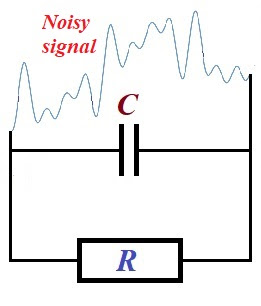The signal (electrical current) coming through a resistor will have higher frequencies weakened by a presence of a capacitor.
Explain why.

Explanation

Reactance of a capacitor XC, which functionally similar to a resistance of a resistor, is inversely proportional to a frequency of the voltage on its ends
XC = 1/(ω·C) = 1/(2πf·C),
where
ω=2πf is the angular velocity of oscillations,
f is a frequency of oscillations,
C is the capacitance of a capacitor.
Therefore, the greater frequency - the less reactance of a capacitor.
Since reactance of a capacitor is functionally similar to a resistance of a resistor, this circuit is similar to a circuit with two parallel resistors with one of them having lower resistance with higher frequencies of the oscillations of the electrical current.

Using this analogy, we see that higher frequency oscillations of the electric current going through a capacitor will meet less resistance than lower frequency oscillations. Therefore, higher frequency oscillations of the current will go easier through a capacitor, thus having less impact on a resistor.

More precisely, the current going through parallel resistors is inversely proportional to their resistance (see lecture "DC Ohm's Law" in this part of the course). This is true not only for constant direct current produced by constant EMF E, but also at any moment t, when generated EMF oscillates (even irregularly) as a function of time E(t).
Therefore, a capacitor will lower reactance will have higher current going through it, thus weakening the higher frequency oscillations of the current going through a resistor.

Problem C

Consider a circuit that contains a source of a noisy electrical signal, which is a combination of many sinusoidal waves with different frequencies, amplitudes and phase shifts, a resistor with resistance R and an inductor with inductance L connected parallel to a resistor.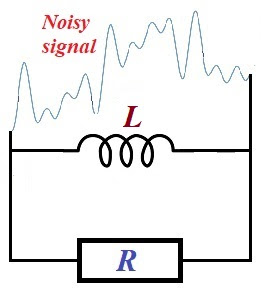The signal (electrical current) coming through a resistor will have lower frequencies weakened by a presence of a inductor.
Explain why.

Explanation

Reactance of an inductor XL, which functionally similar to a resistance of a resistor, is proportional to a frequency of the voltage on its ends
XL = ω·L = 2πf·L,
where
ω=2πf is the angular velocity of oscillations,
f is a frequency of oscillations,
L is the inductance of an inductor.
Therefore, the lower frequency - the less reactance of an inductor.
Since reactance of an inductor is functionally similar to a resistance of a resistor, this circuit is similar to a circuit with two parallel resistors with one of them having lower resistance with lower frequencies of the oscillations of the electrical current.

Using this analogy, we see that lower frequency oscillations of the electric current going through an inductor will meet less resistance than higher frequency oscillations. Therefore, lower frequency oscillations of the current will go easier through an inductor, thus having less impact on a resistor.

More precisely, the current going through parallel resistors is inversely proportional to their resistance (see lecture "DC Ohm's Law" in this part of the course). This is true not only for constant direct current produced by constant EMF E, but also at any moment t, when generated EMF oscillates (even irregularly) as a function of time E(t).
Therefore, an inductor will lower reactance will have higher current going through it, thus weakening the lower frequency oscillations of the current going through a resistor.

SUMMARY
Capacitors and inductors can be used to filter certain "useful" frequencies from the noisy signals.

## Wednesday, October 7, 2020

### Series LC Circuit: UNIZOR.COM - Physics4Teens - Electromagnetism - Alter...

Notes to a video lecture on http://www.unizor.com

Series LC Circuit

Consider a circuit that contains an AC generator, an inductor of inductance L and a capacitor of capacity C in a series.The current IL(t) going through an inductor is the same as the current IC(t) going through a capacitor. So, we will use an expression I(t) for both.

The electromotive force (EMF) generated by an AC generator depends only
on its own properties and can be described as a function of time t

E(t) = E0·sin(ωt)

where

E0 is a peak voltage on the terminals of a generator,

ω is an angular velocity of a rotor in radians per second.

Inductance L of an inductor and capacity C of a capacitor produce voltage drops VL(t) and VC(t) correspondingly.

As we know, the voltage drop on an inductor is causes by self-induction
and depends on the rate of change (that is, the first derivative by
time) of a magnetic flux Φ(t) going through it

VL(t) = dΦ(t)/dt

Magnetic flux, in turn, depends on a current going through a wire of an inductor I(t) and the inductor's inductance L

Φ(t) = L·I(t)

Therefore, the voltage drop on an inductor equals to

VL(t) = L·dI(t)/dt

As we know, the amount of electricity Q(t) accumulated in a capacitor is proportional to voltage VC(t) applied to its plates (that is, voltage drop on a capacitor). The constant capacity of a capacitor C
is the coefficient of proportionality (see lecture "Electric Fields" -
"Capacitors" in this course) that depends on a type of a capacitor

C = Q(t)/VC(t)

Therefore,

Q(t)=C·VC(t)

Knowing the amount of electricity Q(t) accumulated in a capacitor as a function of time t, we can determine the electric current I(t) in a circuit, which is a rate of change (that is, the first derivative by time) of the amount of electricity

I(t) = dQ(t)/dt = C·dVC(t)/dt

The sum of voltage drops on an inductor and a capacitor is supposed to be equal to EMF produced by an AC generator E(t)=E0·sin(ωt), which is the final equation in our system:

VL(t) = L·dI(t)/dt

I(t) = C·dVC(t)/dt

E0·sin(ωt) = VL(t) + VC(t)

To solve this system of three equations, including two differential ones, let's resolve the third equation for VC(t) and substitute it in the second one.

VC(t) = E0·sin(ωt) − VL(t)

I(t)=C·d[E0·sin(ωt)−VL(t)]/dt

In the last equation we can differentiate each component and, using symbol ' for a derivative, obtain

I(t)=CωE0·cos(ωt)−C·V'L(t)

Together with the first equation from the original system of three
equations above we have reduced the system to two equations (again, we
use symbol ' for brevity to signify differentiation)

VL(t) = L·I'(t)

I(t)=CωE0·cos(ωt)−C·V'L(t)

Substituting VL(t) from the first of these equations into the second, we obtain one equation for I(t), which happens to be a differential equation of the second order (we will use symbol " to signify a second derivative of I(t) for brevity)

I(t)=CωE0·cos(ωt)−CL·I"(t)

or in a more traditional for differential equation form

a·I"(t) + b·I(t) = E0·cos(ωt)

where

a = L/ω

b = 1/(Cω)

Without getting too deep into a theory of differential equations, notice that the one and only known function in this equation that depends on time t is cos(ωt). It's second derivative also contains cos(ωt). So, if I(t) is proportional to cos(ωt), its second derivative I"(t) will also be proportional to cos(ωt) and we can find the coefficient of proportionality to satisfy the equation.

Let's try to find such coefficient K that function I(t)=K·cos(ωt) satisfies our equation.

I'(t) = −ωK·sin(ωt)

I"(t) = −ω²K·cos(ωt)

Now our differential equation for I(t) is

−a·ω²K·cos(ωt) + b·K·cos(ωt) = E0·cos(ωt)

From this we can easily find a coefficient K

K = E0/(b−aω²)

Since a=L/ω and b=1/(Cω)

K = E0/{[1/(Cω)] − Lω}

In the previous lectures we have introduced concepts of capacitive reactance XC=1/(Cω) and inductive reactance XL=Lω. Using these variables, the expression for coefficient K is
K = E0/(XC−XL)

Therefore,

I(t) = E0·cos(ωt)/(XC−XL)

or

I(t) = I0·cos(ωt)

where

I0 = E0/(XC−XL)

The last equation brings us to a concept of a reactance of the LC circuit

XC−XL

that is similar to resistance of regular resistors.

Using a concept of reactance, the last equation resembles the Ohm's Law.

Let's determine the voltage drops on a capacitor VC(t) and an inductor VL(t) using the expression for the current I(t).

Since Q(t)=C·VC(t) and I(t)=Q'(t), we can find VC(t) by integrating I(t)/C.

VC(t) = [0,t]I(t)·dt/C =

= I0·sin(ωt)/(C·ω) =

= XC·E0·sin(ωt)/(XC−XL)

VL(t) = L·I'(t) =

= −L·E0·sin(ωt)·ω/(XC−XL) =

= −XL·E0·sin(ωt)/(XC−XL)

Let's check that the sum of voltage drops in the circuit VL(t) and VC(t) is equal to the original EMF generated by a source of electricity.

Indeed,

VL(t) + VC(t) =

=(XC−XL)·E0·sin(ωt)/(XC−XL)=

= E0·sin(ωt)

Summary

EDF generated by a source of electricity

E(t) = E0·sin(ωt)

where

E0 is a peak voltage on the terminals of a generator,

ω is an angular velocity of a rotor in radians per second.

Alternating electric current in the circuit

I(t) = I0·cos(ωt)

where

I0 = E0/(XC−XL)

XC = 1/(ω·C)

XL = ω·L

Voltage drop on a capacitor

VC(t) = XC·E0·sin(ωt)/(XC−XL)

Voltage drop on an inductor

VL(t) = −XL·E0·sin(ωt)/(XC−XL)

Phase Shift

Notice that cos(x)=sin(x+π/2). Graph of function y=sin(x+π/2) is shifted to the left by π/2 relative to graph of y=sin(x).

Therefore, oscillations of the current I(t) in the LC circuit are ahead of oscillation of the EMF generated by a source of electricity E(t) by a phase shift of π/2.

Oscillations of the voltage drop on a capacitor VC(t) in the LC circuit are synchronized (in phase) with generated EMF.

Notice that −sin(x)=sin(x+π). Graph of function y=sin(x+π) is shifted to the left by π relative to graph of y=sin(x).

Therefore, oscillations of the voltage drop on an inductor VL(t) in the LC circuit are ahead of oscillation of the EMF generated by a source of electricity E(t) by π (this is called in antiphase).

## Friday, October 2, 2020

### AC Inductors: UNIZOR.COM - Physics4Teens - Electromagnetism

Notes to a video lecture on http://www.unizor.com

Alternating Current and Inductors

For the purpose of this lecture it's important to be familiar with the concept of a self-induction explained in "Electromagnetism - Self-Induction" chapter of this course.

In this lecture we will discuss the AC circuit that contains an inductor - a wire wound in a reel or a solenoid, thus making multiple loops, schematically presented on the following picture.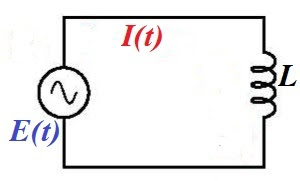Both direct and alternating current go through an inductor, but, while
direct current goes with very little resistance through a wire, whether
it's in a shape of a loop or not, alternating current meets some
additional resistance when this wire is wound into a loop.

Consider the following experiment.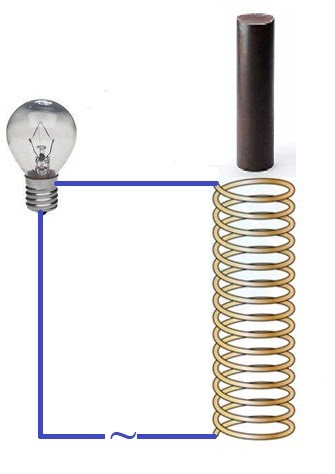Here the AC circuit includes a lamp, an inductor in a shape of a solenoid and an iron rod fit to be inserted into a solenoid.

While the rod is not inside a solenoid, the lamp lights with normal
intensity. But let's gradually insert an iron core into a solenoid. As
the core goes deeper into a solenoid, the lamp produces less and less
light, as if some kind of resistance is increasing in the circuit.

This experiment demonstrates that inductors in the AC circuit produce
effect similar to resistors, and the more "inductive" the inductor - the
more resistance can be observed in a circuit.

The theory behind this is explained in this lecture.

The cause of this resistance is self-induction. This concept was
explained earlier in this course and its essence is that variable
magnetic field flux, going through a wire loop, creates electromotive
force (EMF) directed against the original EMF that drives electric
current through a loop.

Any current that goes along a wire creates a magnetic field around this
wire. Since the current in our wire loop is alternating, the magnetic
field that goes through this loop is variable. According to the
Faraday's Law, the variable magnetic field going through a wire loop
generates EMF equal to a rate of change of the magnetic field flux and
directed opposite to the EMF that drives the current through a wire,
thus resisting it.

Magnetic flux Φ(t) going through inductor, as a function of time t, is proportional to an electric current I(t) going through its wire

Φ(t) = L·I(t)

where L is a coefficient of proportionality that depends
on physical properties of the inductor (number of loop in a reel, type
of its core etc.) called inductance of the inductor.

If the current is alternating as

I(t) = Imax·sin(ωt)

the flux will be

Φ(t) = L·Imax·sin(ωt)

According to Faraday's Law, self-induction EMF Ei
is equal in magnitude to a rate of change of magnetic flux and opposite
in sign (see chapter "Electromagnetism - "Self-Induction" in this
course)

Ei(t) = −dΦ/dt =

= −L·
dI(t)/dt =

= −L·ω·Imax·cos(ωt) =

= −L·ω·Imax·sin(ωt+π/2) =

= −Eimax·sin(ωt+π/2)

where

Eimax = L·ω·Imax

The unit of measurement of inductance is henry (H) with 1H being an inductance of an inductor that generates 1V electromotive force, if the rate of change of current is 1A/sec.

That is,

henry = volt·sec/ampere = ohm·sec

An expression XL=L·ω in the above formula for Ei is called inductive reactance. It plays the same role for an inductor as resistance for resistors.

The units of the inductive reactance is Ohm (Ω) because

henry/sec = ohm·sec/sec = ohm.

Using this concept of inductive reactance XL of an inductor, the time dependent induced EMF is

Ei(t) = −XL·Imax·sin(ωt+π/2) = −Eimax·sin(ωt+π/2)

and

Eimax = XL·Imax,

which for inductors in AC circuit is an analogue of the Ohm's Law for resistors.

What's most important in the formula

Ei(t) = −Eimax·sin(ωt+π/2)

and the most important property of an inductor in an AC circuit is
that, while the electric current in a circuit oscillates with angular
speed ω, the voltage drop on an inductor oscillates with the same angular speed ω as the current, but its period is shifted in time by π/2 relative to the current.

## Tuesday, September 29, 2020

### UNIZOR.COM - Physics4Teens - Electromagnetism - Ohm's Law - Problems 4

Notes to a video lecture on http://www.unizor.com

Direct Current - Ohm's Law - Problems 4

Problem A

Given a circuit presented on a picture below.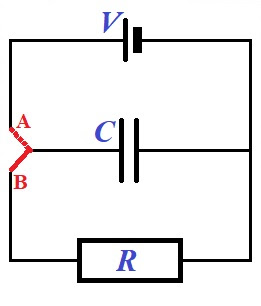Initially, a red switch is in position A to fully charge a capacitor of capacity C from a battery producing a direct current with voltage V.

When a capacitor is fully charged, a switch is moved to position B, disconnecting a capacitor from a battery and forming a new circuit that includes only a fully charged capacitor and a resistor of resistance R.

When a switch is in position B, a
capacitor starts discharging its charge through a resistor. It's charge
will gradually diminish to zero, when all excess electrons on its one
plate will flow through a resistor to a plate with deficiency of
electrons.

During this process of discharge the electric current in a circuit that
contains a capacitor and a resistor will change from some maximum value
in the beginning of this process to zero, when the discharge is
completed.

Find the charge on a capacitor Q(t) and an electric current flowing trough a resistor I(t) as functions of time t.

Solution

Assume, our switch is in position A, and we are at the charging stage, when the battery of voltage V is charging a capacitor of capacity C.

The capacity of a capacitor is defined as the constant ratio of a charge
accumulated by a capacitor to a voltage applied to its plate (see
"Capacitors" lecture in the "Electromagnetism - Electric Field"
chapter):

C = Q/V

Therefore, the full charge of a capacitor at the end of the first stage of charging is V·C.

Then we flip a switch into position B, starting the second stage - discharging of a capacitor through a resistor.

At the beginning of this second stage a capacitor is fully charged. So, at time t=0 its charge is

Q(0) = V·C

The charge on a capacitor at any time produces a voltage between its plates

V(t) = Q(t)/C

This voltage produces a current flowing through a resistor I(t) that, according to the Ohm's Law, should be equal to

I(t) = V(t)/R

From the two equations above we conclude

Q(t)/C = I(t)·R

This is our first equation that connects two time-dependent (that is,
functions of time) variables - an electric current in a circuit I(t) and a charge on a capacitor Q(t).

The second functional equation is, basically, a definition of an
electric current as the rate of electric charge flowing in a circuit
(that is, amperage is how much electricity in coulombs flows through a circuit per unit of time - a second).

Mathematically speaking, an electric current is the first derivative of
an electric charge by time, taken with a sign that depends on the
direction of the change of the charge (plus if the charge is increasing and minus if decreasing):

I(t) = −dQ(t)/dt

Considering the charge Q(t) is decreasing and, therefore,
its derivative is negative, while we would like the electric current to a
be a positive number, we have to use a minus sign in this equation.

This is our second functional equation (that happens to be differential)
connecting two functions - an electric current in a circuit I(t) and a charge on a capacitor Q(t).

Now we have two functional equations, one of them is differential, and an initial condition:

Q(t)/C = I(t)·R

I(t) = −dQ(t)/dt

Q(0) = V·C

It's up to our mathematical skills to solve this system of equations.

First, we substitute I(t) from the second equation into the first, getting a differential equation for Q(t)

Q(t)/C = −R·dQ(t)/dt

This can be converted into

dQ(t)/Q(t) = −dt/(R·C)

or

d[ln(Q(t))] = d[−t/(R·C)]

If differentials of two functions are equal, the functions themselves
are just separated by a constant that can be determined using the
initial condition. Let denote that constant as K.

ln(Q(t)) = −t/(R·C) + K

or, applying an exponent to both sides of this equation,

Q(t) = eK·e−t/(R·C)

It's time to use the initial condition Q(0)=V·C to determine the multiplier eK.

For t=0 the right side of an expression for Q(t) equals to eK. Therefore, this multiplier equals to V·C.

Therefore, the final expression for a charge on a capacitor as a function of time Q(t) is

Q(t) = V·C·e−t/(R·C)

So, a charge on a capacitor is exponentially diminishing.

From the expression of Q(t) we can find the expression on an electric current going through a resistor, using the equation

I(t) = −dQ(t)/dt

from which follows

I(t) = −d[V·C·e−t/(R·C)]/dt =

= −V·C·
d
[e−t/(R·C)]/dt =

= −(V·C)·(−1/(R·C))·e−t/(R·C) =

= (V/R)·e−t/(R·C)

Q(t) = (V·C)·e−t/(R·C)

The multiplier V·C is the initial full charge of a capacitor.

I(t) = (V/R)·e−t/(R·C)

The multiplier V/R is the current that would flow through a resistor, if there were no capacitor. This follows from the Ohm's Law.